and ortutorials

# interferences

Basics

Interferences occur when the luminous intensity resulting from the superposition of several light waves is different from the sum of the light waves intensities. Interferences are characteristic of the wavelike behaviour of light. Interferences intensity depends on phase shift of the different superimposed light waves and requests some conditions. Interfering light waves must propagate in close enough directions and must have close enough wavelengths. Light waves must also be synchronised, which requests that in most cases, they come from a unique source or at least synchronized sources. When emitted by the same light source, interfering waves are synchronized when the delay between their emission is smaller than a parameter called the coherence time of the light source. Coherent time defines another parameter called the coherence length, which is the maximum optical distance separating possibly interfering photons. Coherent time and length depend on spectrum width. They increase when spectrum width decreases. A unique light source must be small enough for allowing interferences. This condition is called the spatial coherence.

A particular case concerns interferences between two propagating monochromatic isochromic waves. If interferences occur at a point, the variable term of intensity is a cosine function of the phase shift between the two waves at this point. This phase shift is constant with time. This means that average intensity is maximum when phase shift is 2 p p ( constructive interference ) and minimum when phase shift is ( 2p+1 ) p ( destructive interference ), p being an integer . p is called the interference order.

Interferences with plane waves

It is supposed that waves are plane monochromatic, isochromic and synchronized. This can be the case for a single monochromatic ponctual light source located at infinity. Different types of interferences can occur.

In the first type, light wave is separated in two plane waves ( w1 and w2 ) propagating in two different directions and then recombined. Intensity is then constant in every plane perpendicular to the propagation direction. It depends on phase shift between w1 and w2 depending itself on difference between optical paths covered respectively by w1 and w2.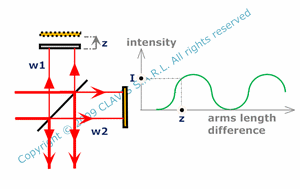This concerns ( not exclusively ) Michelson interferometer with parallel mirrors. This interferometer is made of a beam splitter, so that part w1 of the wave is reflected to a mirror M1, the other part w2 being transmitted to another mirror M2. After reflection on the respective mirrors M1 and M2, w1 and w2 are recombined by the beam splitter ( which at this moment becomes a beam combiner ). Phase shift is function of the wavelength ( lam ) and the difference between the optical paths covered respectively by W1 and w2. This optical paths difference depends on mirrors relative positions expressed by the difference ( z ) between arms lengths of the interferometer. When arms lengths are equal ( z=0 ), interferences intensity is minimum because of the different reflection types produced by the beam splitter for w1 and w2 ( glass/air reflection for one wave and air/glass reflection for the other wave ) generating a phase shift of p. Interferences can't occur if the difference between optical paths is larger than the coherence length of the light source.In a second type of interferences ( air corner interferences ), part w1 of the incident light wave is reflected by a first plane surface S1 when part w2 of the transmitted light wave is reflected by a second plane surface S2 unparallel to the first one. S1 and S2 are separated by air. It is assumed that incident plane is perpendicular to the straight line O, intersection of the two surfaces. w1 and w2 propagate with different directions. The angle between the surfaces is small enough to allow interferences between w1 and w2. The interfringe dx depends on the orientation of the observer plane. When the observer plane is parallel to the first surface S1, dx depends on the wavelength ( lam), alfa ( angle between the surfaces ) and i ( angle of incidence on the first surface). When the observer plane is perpendicular to equiphase planes, dx depends on lam and alfa.This second type of intereferences concerns also a Michelson interferometer with non perpendicular mirrors ( angle between mirrors being 90°+alfa ). In this case, the incident light wave is separated by the beam splitter in two waves reflected by the respective mirrors M1 and M2 in slightly different directions. Using conjugation of mirror M2 by the beam splitter gives a system equivalent to an air corner where surfaces are replaced by mirrors with an angle alfa, the angle of incidence being null.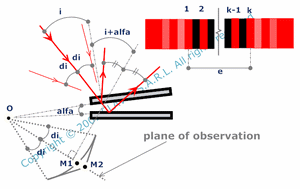In the case of an extended light source, light rays do not all have the same direction. Equiphase planes are different depending on the angle of incidence and interference are blurred. However, in an observation plane P passing through the point O intersection of the two reflective surfaces, the gap between equiphase planes is minimum and the fringes can be observed in a certain limit. Fringes can be visible around any point M1 of the observation plane if the distance M1M2 is less than x/4, x being the interfringe at the angle of incidence i. M2 is the intersection of the planes tangent to the circle with center O and passing through M1 but also perpendicular to the equiphase planes corresponding to i-di and i+di.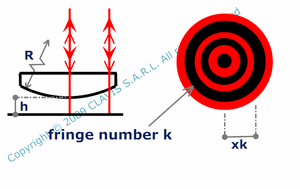In a third type of interferences, two waves recombine by reflection of an incident plane wave on two surfaces ( interfaces ). These surfaces are not necessarily plane and can be considered as a succession of small air corners. Interferences are then localized close to the surfaces. A particular case concerns interferences produced by a spherical interface and a plane interface at normal incidence. The interferences pattern is then made of circular bright and dark bands ( ring finger fringes ) centered on the vertex of the spherical surface. The radius ( xk ) of a ring finger fringe depends on its number ( k ) calculated from the center, the wavelength ( lam ), the gap between the plane and spherical interfaces ( h ) and the radius of the spherical surface ( R ).
In the calculations, both surfaces where reflection occur are supposed to be dielectric interfaces. Consequently there is an air/glass and a glass/air reflection introducing an additionnal phase shift of p.

In général, the number k of a fringe is different from its order.

Interferences produced by a plane parallel plate with diverging waves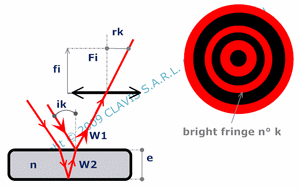It is assumed that the incident wave is monochromatic. The light source located at infinity is not ponctual and generates a diverging light wave ( all light rays don't have the same direction ). Part w1 of the light wave is reflected by a first plane interface when part w2 of the transmitted light wave is reflected by a second plane interface ( parallel to the first one ). w1 and w2 propagate in the same direction and produce interferences. Because the phase shift between w1 and w2 is constant for light rays with the same inclination, interferences are located at infinity and intensity is constant for a given angle of incidence. This means that the interference pattern can be observed in the focal plane of a lens and is made of circles with different intensities, including dark and bright circles ( Newton rings ) corresponding to angles of incidence generating respectively destructive and constructive intereferences. Angles of incidence ik corresponding to these destructive/constructive interferences depend on the wavelength lam, the interferometer thickness e, the refraction index ( n ) of the material in between the two interfaces and the fringe number k. k is the number of dark/bright fringes from the center up to the considered dark/bright fringe. When observed in the focal plane of a lens with an effective focal length fi, the radius Rk of a the dark/bright fringe depends on fi, e, n and k.

In this case also, the number k of a fringe is different from its order.

Fabry Perot interferometerFabry Perot interferometer is made of two parallel interfaces and is generating interferences by transmission. Unlike precedent paragraphs, Fabry Perot doesn't produce two waves interferences but multiwaves interferences. Considering an incident monochromatic plane wave w with an angle of incidence i, part of the wave wo is directly transmitted by the Fabry Perot. Part of the wave w1 results from one "round trip" in the Fabry Perot which means that it is part of the wave transmitted by the first surface, then reflected by the second surface, then reflected by the first surface and finally transmitted by the second surface. w2 results from two round trips in the Fabry Perot, , wn results from n round trips in the Fabry Perot, etc Wave transmitted by the Fabry Perot is the combination of wo, w1, w2, , wn

The relative intensity ( or transmission T ) of this transmitted wave depends on the wavelength ( lam ), the refraction index ( n ) of the material between the interfaces, the Fabry Perot thickness ( e ), the reflectivity coefficient of each interface ( R ) and the angle of incidence ( i ). All these parameters being fixed except lam, the maximum of transmission is achieved for lam giving a phase shift on a round trip of 2 k p, k being an integer ( consequently, e must be larger than lam ). The value of this maximum of transmission is 1. Transmission versus frequency is a periodic curve with a constant pitch between maxima. This pitch is called the free spectral range ( FSR ) of the Fabry Perot for the given incidence angle i. Frequencies for which the transmission is maximum are the resonant frequencies or modes of the Fabry Perot. The finesse of the Fabry Perot is the ratio between the FSR and the linewidth ( FWHM ) of a mode. The larger is R, the larger is the finesse which means that the more resonant are the modes. The theoretical limit (never achieved experimentally ) is for R = 1 where transmission is 1 for modes, 0 for other frequencies and for which finesse is infinite. Rmax is the maximum ratio of light reflected by the Fabry perot and is obtained for non resonant frequencies. Because the spectrum is often given in wavelengths unit, it is practical to give Fabry Perot transmission ( T ) as a function of wavelength and calculate the free spectral range ( SWR ) in the wavelength space around a given wavelength ( lam ), the closest resonant wavelength ( lamFP ) from lam and its linewidth ( dlam). It can also be useful to calculate a parameter ( like i, n, e or the wavelength ) knowing the Fabry Perot transmission ( T ) and the other parameters.When illuminated by a monochromatic extended source, the Fabry Perot generates multiwaves ring interference fringes located at infinity. Each ring corresponds to a given angle of incidence on the Fabry Perot and has a given intensity. The larger is R, the larger is the finesse and the thinner are the bright ring fringes.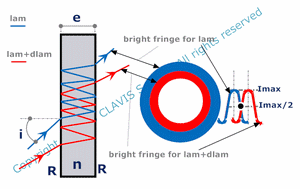When illuminated by a polychromatic extended light source, the Fabry perot acts as a spectrometer by generating set of bright rings with radii depending on the wavelength. Two parameters can be then defined : the phase dispersion Pd which is the derived function of the phase with respect to the wavelength and the power of resolution Pr which is the ratio of the wavelength on the minimum variation of wavelength distinguishable.

References

"Optique Fondements et applications" - 2004 - author : José-Philippe Perez.

"Cours d'optique physique" - Institut d'optique théorique et appliquée - 1985 - author : Christian Imbert.

"Cours d'optique ondulatoire" - Université Denis Diderot Paris 7 - 2006 - author : G.Rebmann.

"Études graphiques des propriétés optiques des lames minces" - Journal de physique - 1950 - author : D. Malé.